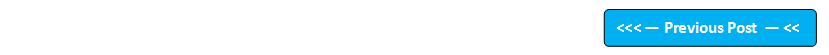## Blog Pages

### 54. 'if' selection statements'if' selection statements are used for the below reason -

If you want any statement to be executed only when the condition passes. Then we have to go with if selection statements.  i.e. Lets say there is a software at park entrance, which says -> for children below 10 years they charge entrace ticket at Rs 50 and for others its free. So in this case we can use if selection statement, when age less than 10 years is true, Rs 50 should be assigned to the ticket price. if selection statement is one of the 'Control Flow' Statements.

 Program to demonstrate the if condition statement when the condition provided to it results true class IfConditionTrue {    public static void main(String args[])    {       int a=3,b=4;            if(a if(3<4) -> if(true)            {                 System.out.println("You are Lucky");             }    }  } Output of this Program: You are Lucky As the result of the condition provide in the if statement i.e. a if(4<3) -> if(false)            {                System.out.println("You are lucky");             }     }  } Output of this program:  (No Output) The program runs without any errors but nothing will get printed as the condition provided in the if statement i.e. ay)      {         System.out.println("x is greater than y");        }        if(x==y)       {         System.out.println("x is equal to y");        }    }  } Output of this program: x is equal to y If.....else... Statement  (program demonstration) /* As you have seen in the above programs, that the statements inside the if block wont execute if the conditions fails, and hence the program wont print anything. But we can add addition else block to the if block, so that the program can execute the statements inside the else block if the if block condition fails. */ class IfElse {    public static void main(String args[])    {       int x=4,y=3;        if(xc)                  System.out.println(" I know this text in the inner 'if' block is not going to be printed on execution as the condition of this inner 'if' block results fail");               else                  System.out.println("We are inside the inner 'else' block");         }         else            System.out.println("I know this text in the outer 'else' block is not going to be printed on execution as the condition of the inner 'if' block results pass");     }  } Output of this program: We are inside the Outer 'if' block  We are inside the inner 'else' block if-else-if Ladder (Program demonstration) As you can see in our previous program where we have executed the 'if' block if the condition results true and executing the 'else' block without any condition. But in this if-else-if ladder, we will add condition to the 'else' part until all our conditions gets finished. class IfElseIf {    public static void main(String args[])    {         int a=3,b=4,c=5;         if(a==4)            System.out.println("This will get printed if the a==4 condition resutls ture, else it will check for the next condition in the else-if part");         else if(b==5)           System.out.println("This will get printed if the b==5 condition results true, else it will check for the next condition in the else-if part");        else if(c==6)            System.out.println("This will get printed if the c==6 condition results true, else it will check for the next condition in the else-if part");          else            System.out.println("All the above conditions failed, hence this statement got executed and printed");     }  } Output of this program: All the above conditions failed, hence this statement got executed and printed Lets go through the program once: started with if(a==4) i..e. 3==4 i.e. false, hence the statement inside the 'if' block didn't execute. (The program would have ended if  if(a==4) resulted true) Next it executed else if(b==5) i.e. b==5 -> 4==5 -> false. Hence the statement inside else if(b==5) didn't execute. (The program would have ended if else if(b==5) resulted true) Next it executed else if(c==6) i.e. c==6 -> 5==6 -> false. Hence the statement inside else if(c==6) didn't execute (The program would have ended if else if(c==6) resulted true) Next it executed 'else' block and printed "All the above conditions failed, hence this statement got executed and printed"